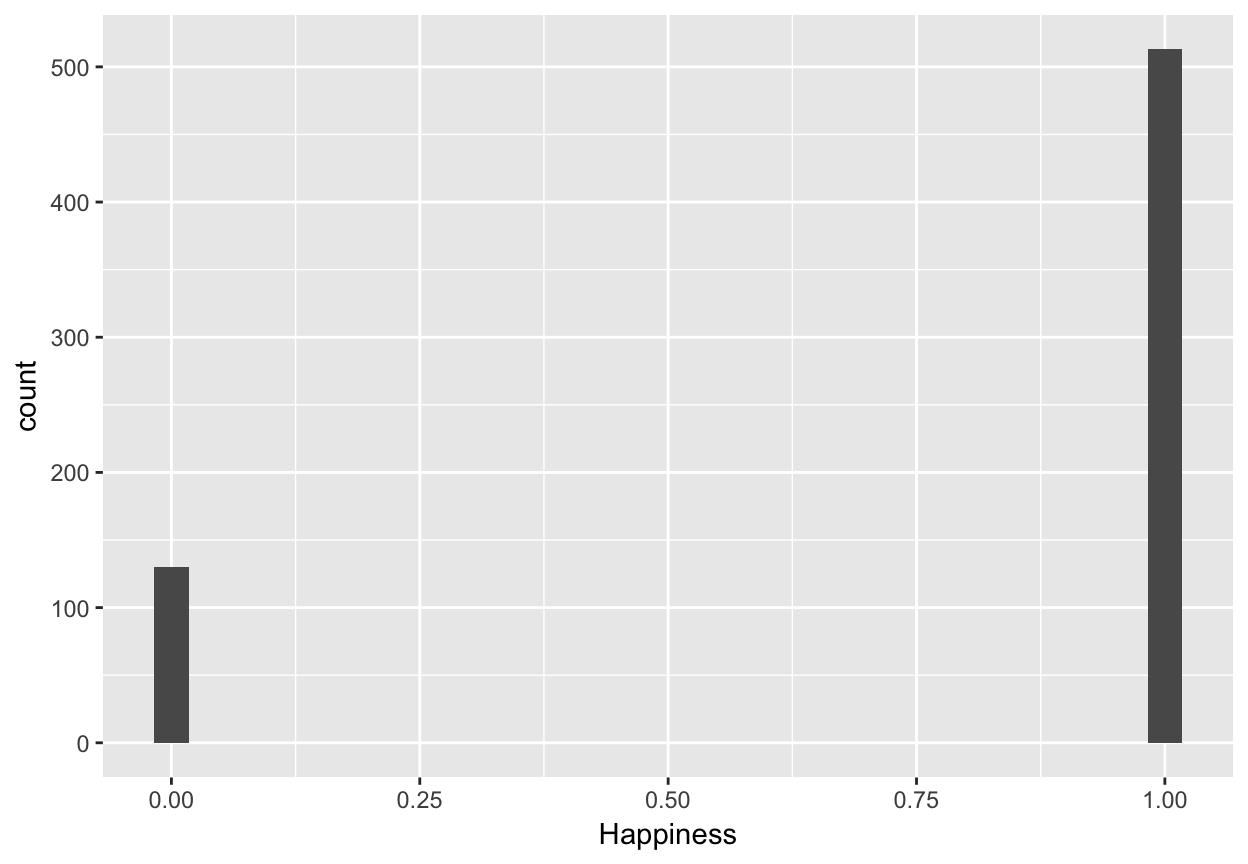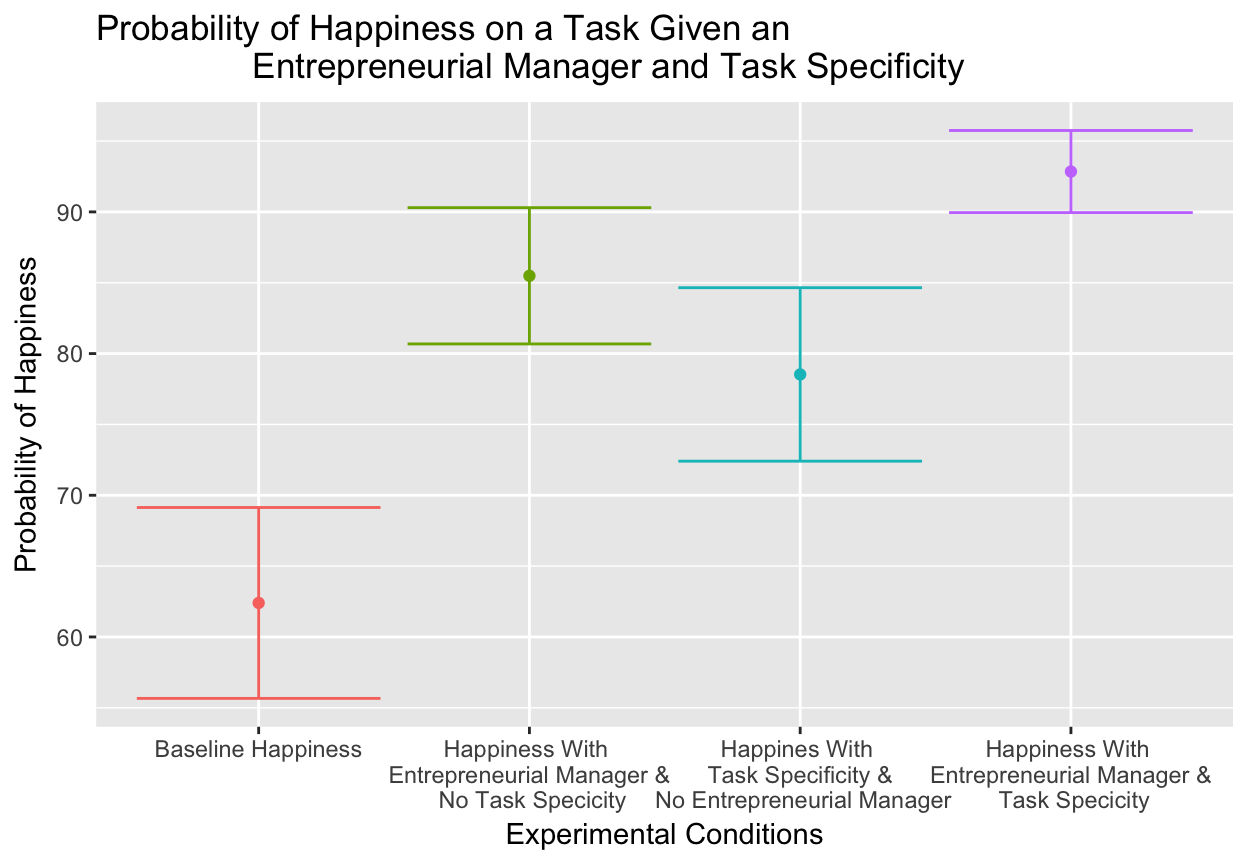Assistant Professor | Bloch School of ManagementPlan of the day

• Project briefing
• Experiment debrief
• Basics of limited dependent variable models
• Quiz

Please welcome Cyrus Hund, JE Dunn Construction.

Lets take a look at the final deliverable.

Experiment debrief…

What was the carryover effect in the experiment, and why would it matter?

What is the benefit of pre-registering an experiment again?

GO BRONCOS!!!

library(tidyverse)
experiment.df <- as.data.frame(dsom.ds)
##   Happiness Creativity EntManager TaskSpecificity RespAge RespExper
## 1         1          5          0               1      39       0.2
## 2         1          6          1               1      39       3.5
## 3         0          5          0               0      33       3.5
## 4         1          3          1               1      44       5.2
## 5         1          5          1               0      40       3.1
##   RespGender Obs
## 1          0   1
## 2          1   2
## 3          1   3
## 4          0   4
## 5          0   5

Our multiple limited dependent variable model for today…

$$Happiness=\alpha+\beta{EntManager}+\beta{TaskSpecificity}+\epsilon$$

Limited dependent variable models encompass a class of estimators where the dependent variable is censored in some nominal way.

Usually in these models, the DV is dichotomous (0 or 1), or categorical (1 = Joint venture, 2 = Acquisition, 3 = Strategic partnership, etc.).

With a censored dependent variable (0, or 1 for example), we can’t use regular OLS regression, because it violates one of the key assumptions—$$i.i.d.$$ being one—and the estimates it produces would be uninterpretable.

qplot(Happiness, data = experiment.df)The solution for a dichotomous outcome is to use a transformation of the OLS regression model, called a ‘logit’ model.

There’s another model called ‘probit’ that works is a very similar way, and the choice is really one that you are most comfortable with.

I think it’s easier to interpret the results of a logit model, so that’s my ‘go-to’ for testing hypotheses with dichotomous dependent variables.

As we will see, rather than explaining variance in the DV, in a logit model, we’re predicting the ‘log odds’ that the DV will happen (the $$y$$ = 1 condition).

The log odds are the natural log of the odds, and the odds is the probability that an event will occur divided by the probability that the event will not occur.

We use the odds ratio—the difference in the odds between two groups—to derive the difference in the probability, and hence, the likelihood of observing the effect we are modeling ($$y$$ = 1).

It may seem complicated, but…

The cool thing is that a logistic regression model is simply a logistic transformation of the linear model, so we can write our model in a familiar form:

$$ln\left[\frac{p}{1-p}\right]=\alpha+{\beta}{x_1}+{\beta}{x_2}+\epsilon$$

Our outcome variable is the log odds of $$y$$ occurring (y = 1), which we write as $$\left[\frac{p}{1-p}\right]$$, the probability of $$y$$ occurring divided by the probability of $$y$$ not occurring.

Here’s the material point…

With a logit model, we’re really interested in the probability that an event (our $$y$$ = 1 condition) will occur, as a function of a change in our independent variable(s).

Because we’re modeling probabilities, it makes logit models a very powerful tool for business.

Lets do a model. We'll use the glm function, and specify the binomial link function, which gives us our logistic transformation.

happiness.logit <- glm(Happiness ~ EntManager + TaskSpecificity,
data = experiment.df, family = "binomial")
summary(happiness.logit)
##
## Call:
## glm(formula = Happiness ~ EntManager + TaskSpecificity, family = "binomial",
##     data = experiment.df)
##
## Deviance Residuals:
##     Min       1Q   Median       3Q      Max
## -2.2969   0.3852   0.5599   0.6953   0.9712
##
## Coefficients:
##                 Estimate Std. Error z value Pr(>|z|)
## (Intercept)       0.5066     0.1465   3.458 0.000544 ***
## EntManager        1.2670     0.2192   5.780 7.48e-09 ***
## TaskSpecificity   0.7902     0.2131   3.708 0.000209 ***
## ---
## Signif. codes:  0 '***' 0.001 '**' 0.01 '*' 0.05 '.' 0.1 ' ' 1
##
## (Dispersion parameter for binomial family taken to be 1)
##
##     Null deviance: 647.38  on 642  degrees of freedom
## Residual deviance: 592.11  on 640  degrees of freedom
## AIC: 598.11
##
## Number of Fisher Scoring iterations: 4

The default output for glm is a little hard to read. Lets break it down using the broom package.

library(broom)
glance(happiness.logit)
##   null.deviance df.null    logLik      AIC     BIC deviance df.residual
## 1      647.3801     642 -296.0553 598.1106 611.509 592.1106         640

In an logit model, there is no such thing as $$R^2$$, so we need to use alternate fit indices.

Lets take a look at the estimates that a logit model spits out…

happiness.logit.coef <- tidy(happiness.logit, conf.int = TRUE)
happiness.logit.coef <- happiness.logit.coef %>%
mutate_if(is.numeric, funs(round(., 3)))
happiness.logit.coef
##              term estimate std.error statistic p.value conf.low conf.high
## 1     (Intercept)    0.507     0.146     3.458   0.001    0.222     0.797
## 2      EntManager    1.267     0.219     5.780   0.000    0.846     1.707
## 3 TaskSpecificity    0.790     0.213     3.708   0.000    0.378     1.215
##              term estimate std.error statistic p.value conf.low conf.high
## 1     (Intercept)    0.507     0.146     3.458   0.001    0.222     0.797
## 2      EntManager    1.267     0.219     5.780   0.000    0.846     1.707
## 3 TaskSpecificity    0.790     0.213     3.708   0.000    0.378     1.215

We will get to interpreting the coefficient estimate in a bit. A couple of things with a logit model though…

• The 'statistic' above is actually a $$z$$ statistic, not a $$t$$ statistic. It's still calculated as the coefficient estimate divided by the standard error, but it follows a normal distribution.

• The c.i. values are the margin of error around our coefficient estimate.

##              term estimate std.error statistic p.value conf.low conf.high
## 1     (Intercept)    0.507     0.146     3.458   0.001    0.222     0.797
## 2      EntManager    1.267     0.219     5.780   0.000    0.846     1.707
## 3 TaskSpecificity    0.790     0.213     3.708   0.000    0.378     1.215

Our predicted $$Happiness$$ odds are on the logit scale. That means that the coefficient estimates are the log odds of $$Happiness$$ occurring given the presence of there being an Entrepreneurial Manager versus there not being an Entrepreneurial Manager, and similarly for Task Specificity.

The intercept in this case is the log odds of $$Happiness$$ occurring when both $$EntManager$$ and $$TaskSpecificity$$ are zero.

Log odds are not particularly helpful, so we can convert them from the logit scale by taking the exponent:

happiness.logit.exp <- happiness.logit.coef %>%
dplyr::select(term, estimate) %>%
mutate(odds.ratio = exp(estimate))
happiness.logit.exp
##              term estimate odds.ratio
## 1     (Intercept)    0.507   1.660303
## 2      EntManager    1.267   3.550186
## 3 TaskSpecificity    0.790   2.203396

The odds are 3.56 to 1 that people in the $$EntManager$$ = 1 condition will be Happy compared to the $$EntManager$$ = 0 condition.

The actual value is the expected change in the odds ratio given the presence of an Entrepreneurial Manager, holding constant the impact of Task Specificity.

Quick note, but REALLY important…

If you see an odds ratio below 1.0, that means that the odds of what you are predicting to happen ($$y$$ = 1) actually went down with an increase in your independent variable.

You can never have a negative odds ratio, because you can never have a negative probability.

If the odds ratio then was 1.0, you would have an equivalent probability (a 50/50 chance) of the $$y$$ = 1 condition occurring.

In this case, there would be no meaningful difference in the probability of the $$y$$ = 1 condition occurring as a function of a change in the level of our independent variable.

##              term estimate std.error statistic p.value conf.low conf.high
## 1     (Intercept)    0.507     0.146     3.458   0.001    0.222     0.797
## 2      EntManager    1.267     0.219     5.780   0.000    0.846     1.707
## 3 TaskSpecificity    0.790     0.213     3.708   0.000    0.378     1.215

Once again, our log odds ratios look like they are different from each other, but are they are statistically different from each other?

What do we use here again?

$$H_0:\mu_{EntManager}-\mu_{TaskSpecificity}=0$$

library(multcomp)
creativity.contrast <- glht(happiness.logit, linfct = c("EntManager - TaskSpecificity = 0"))
summary(creativity.contrast)
##
##   Simultaneous Tests for General Linear Hypotheses
##
## Fit: glm(formula = Happiness ~ EntManager + TaskSpecificity, family = "binomial",
##     data = experiment.df)
##
## Linear Hypotheses:
##                                   Estimate Std. Error z value Pr(>|z|)
## EntManager - TaskSpecificity == 0   0.4768     0.3097    1.54    0.124
## (Adjusted p values reported -- single-step method)

So how do we interpret this contrast?

So (log)odds ratios are handy, but like I said before, we are really interested in probabilities, and they are easier to understand.

In our model, given that both $$EntManager(x1)$$ and $$TaskSpecificity(x2)$$ are dichotomous, we have four different possible probabilities of $$Happiness(y)$$ predicted by our model, each of which are below:

Probability x1 x2 Equation
Pr_y 0 0 $$ln\left[\frac{p}{1-p}\right]=\alpha$$
Pr_y 1 0 $$ln\left[\frac{p}{1-p}\right]=\alpha+{\beta}{x_1}$$
Pr_y 0 1 $$ln\left[\frac{p}{1-p}\right]=\alpha+{\beta}{x_2}$$
Pr_y 1 1 $$ln\left[\frac{p}{1-p}\right]=\alpha+{\beta}{x_1}+{\beta}{x_2}$$

We are going to use an R shortcut to estimate these probabilities. If you are interested in the long-way (and it is pretty cool), you can check out my website.

We're going to start by creating a dataframe that mimics the table we setup earlier.

predictor.values <- data_frame(EntManager = c(0, 1, 0, 1),
TaskSpecificity = c(0, 0, 1, 1))
predictor.values
## # A tibble: 4 x 2
##        <dbl>           <dbl>
## 1          0               0
## 2          1               0
## 3          0               1
## 4          1               1

We're going to make use of the broom package again to create a set of predicted probabilities by passing our predictor.values dataframe to our model. The type.predict = "response" option makes sure that we get probabilities and not log odds ratios.

library(broom)
happiness.predict <- broom::augment(x = happiness.logit,
newdata = predictor.values, type.predict = "response")
happiness.predict
##   EntManager TaskSpecificity   .fitted    .se.fit
## 1          0               0 0.6240016 0.03436921
## 2          1               0 0.8549001 0.02453673
## 3          0               1 0.7852920 0.03123567
## 4          1               1 0.9284932 0.01478050

Now lets create our confidence intervals around each probability (the .fitted column in the happiness.predict dataframe), and we'll convert these to percentages to make them easier to interpret:

happiness.predict <- happiness.predict %>%
mutate(Pr_y = 100 * .fitted,
lower.ci = 100 * (.fitted - (1.96 * .se.fit)),
upper.ci = 100 * (.fitted + (1.96 * .se.fit))) %>%
dplyr::select(EntManager, TaskSpecificity, Pr_y, lower.ci, upper.ci)
happiness.predict
##   EntManager TaskSpecificity     Pr_y lower.ci upper.ci
## 1          0               0 62.40016 55.66380 69.13653
## 2          1               0 85.49001 80.68081 90.29921
## 3          0               1 78.52920 72.40701 84.65139
## 4          1               1 92.84932 89.95234 95.74630

Lastly, lets put it together for a visualization. We're going to need to create a new x-axis placeholder to construct our plot, based on our different conditions:

happiness.predict <- happiness.predict %>%
mutate(condition = 1:n())
happiness.predict$condition = factor(happiness.predict$condition)
happiness.predict
##   EntManager TaskSpecificity     Pr_y lower.ci upper.ci condition
## 1          0               0 62.40016 55.66380 69.13653         1
## 2          1               0 85.49001 80.68081 90.29921         2
## 3          0               1 78.52920 72.40701 84.65139         3
## 4          1               1 92.84932 89.95234 95.74630         4
predict.plot <- ggplot(happiness.predict, aes(condition, Pr_y, colour = condition)) +
geom_point() +
geom_errorbar(aes(ymin = lower.ci, ymax = upper.ci)) +
scale_x_discrete(labels=c("Baseline Happiness",
"Happiness With \n Entrepreneurial Manager & \n No Task Specicity",
"Happines With \n Task Specificity & \n No Entrepreneurial Manager",
"Happiness With \n Entrepreneurial Manager & \n Task Specicity")) +
labs(title = "Probability of Happiness on a Task Given an
Entrepreneurial Manager and Task Specificity",
y = "Probability of Happiness",
x = "Experimental Conditions") +
scale_colour_discrete(guide = FALSE)
predict.plotTRAINING TIME OUT

Quiz time!

Lets take a look at the homework.

By the way, you are probably going to want to work this out together as a team, but send me an individual markdown document please.

Wrap-up

What's next?

• Next Class: More on limited dependent variable models
• Deliverables: Draft Presentation // Homework 9 Due 9:00AM Tuesday